Applicable plans
Sprout Blossom Garden EstateForest

Formula fields are auto-calculated fields that derive their value from an expression assigned by the admin. You can create formula fields across all modules (Lead, Contact, Account, and Deal), with a limit of 5 formula fields per module.

1. Defining a formula

When creating a formula field, you need to add the formula using which the field value is calculated. A formula can have four elements to it:

Function: An expression that defines a relationship between one independent variable and the other. Example: sum(5, 10) where sum is a function.

Operator: The symbol indicating an operation to be performed. Example: 5+3=8, where the operator + indicates addition.

Field: This refers to the default fields in the chosen module. The value from the chosen field will be used as a variable in the formula.

Return type: The type of value that will be returned when the formula is computed.

 Note: The number of fields you’ve chosen for formula fields is deducted from the total number of field limits available to you. So if you’re on the Blossom plan and have a maximum limit of 20 number fields, you will now have 15 remaining.

# 2. Creating a formula

Depending on the module you choose and the formula you want to create, you can create a formula as a combination of functions, operators, and fields. Let’s look at an example:

Calculating the commission value for a salesperson based on the deal value

Let’s consider that the commission value for a salesperson is 5% of the deal value. So we’ll create the formula in the deal module, to access the “Deal value” field.

Step 1: Adding a formula field

Go to Admin Settings > Leads/Contacts/Accounts/Deals Module and click thebutton. This brings up the Add Field overlay. Select the ‘Formula’ icon from the custom field tray and click.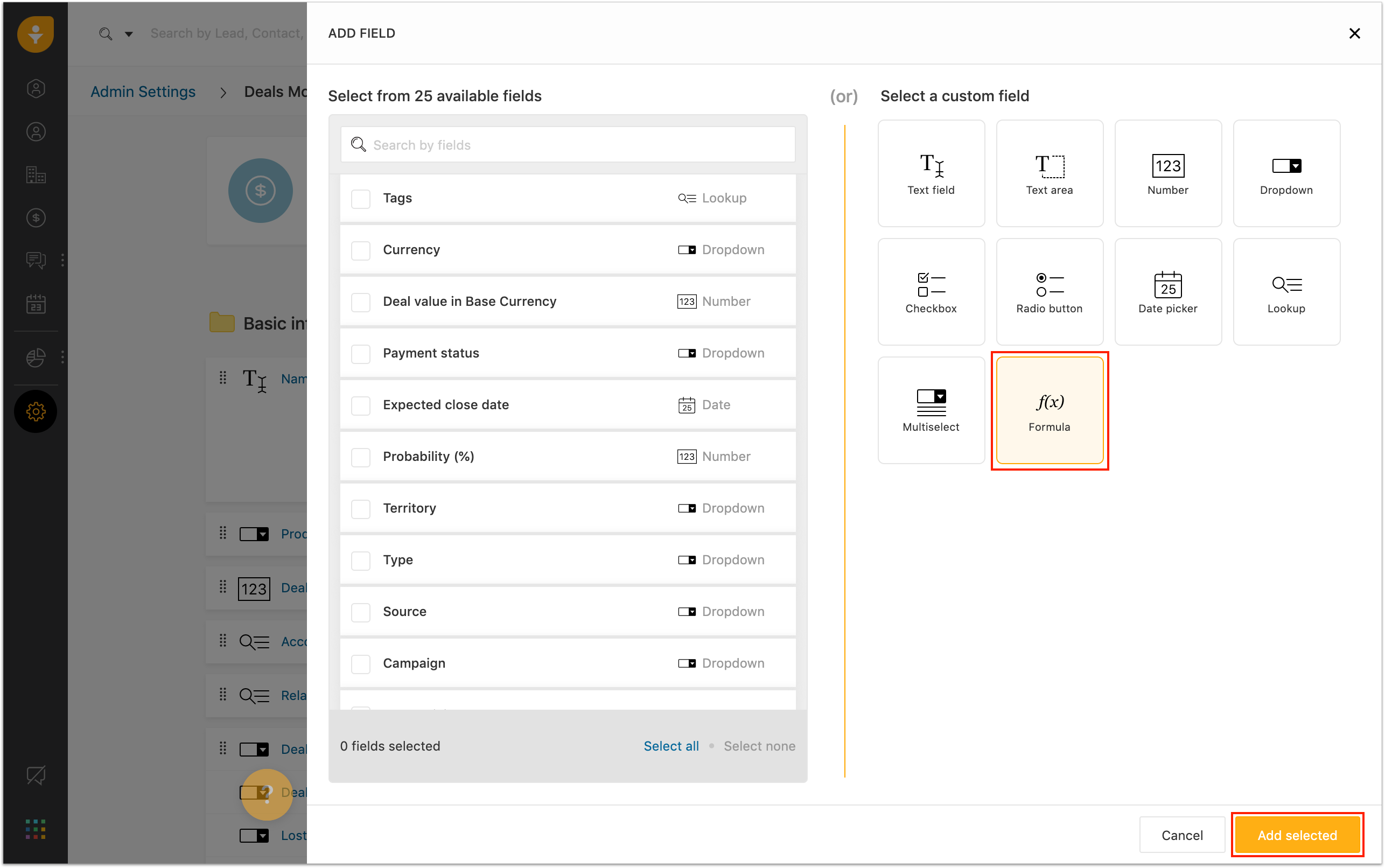Step 2: Name the field and set the return type

Assign your field a name that indicates what the field value is. In this example, we’ve given our field the name, “Commission based on deal value.”

Step 3: Set the return type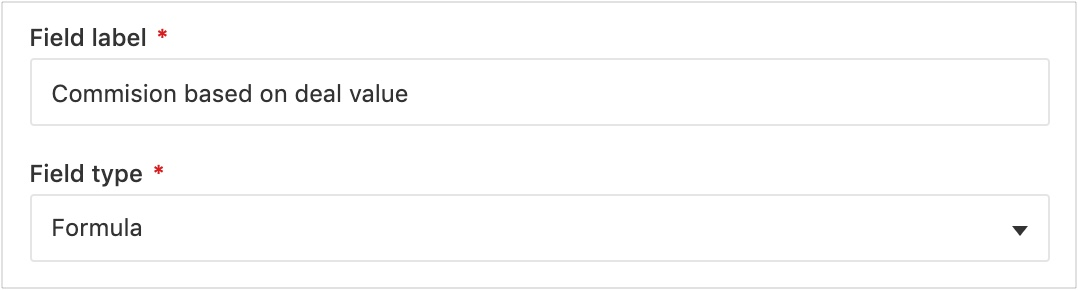Depending on the value that is being calculated, choose the return type of the output from the drop-down. As we’re dealing with commission values in this example, the return type is set as “Number.”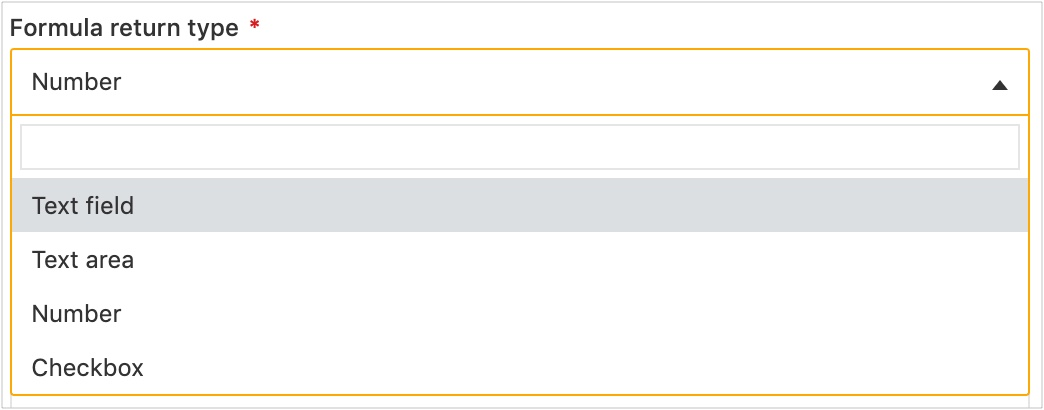Step 4: Pick the formula entities

Here, we use an operator and the deal field. So our formula would be Formula = (5/100) * Deal value.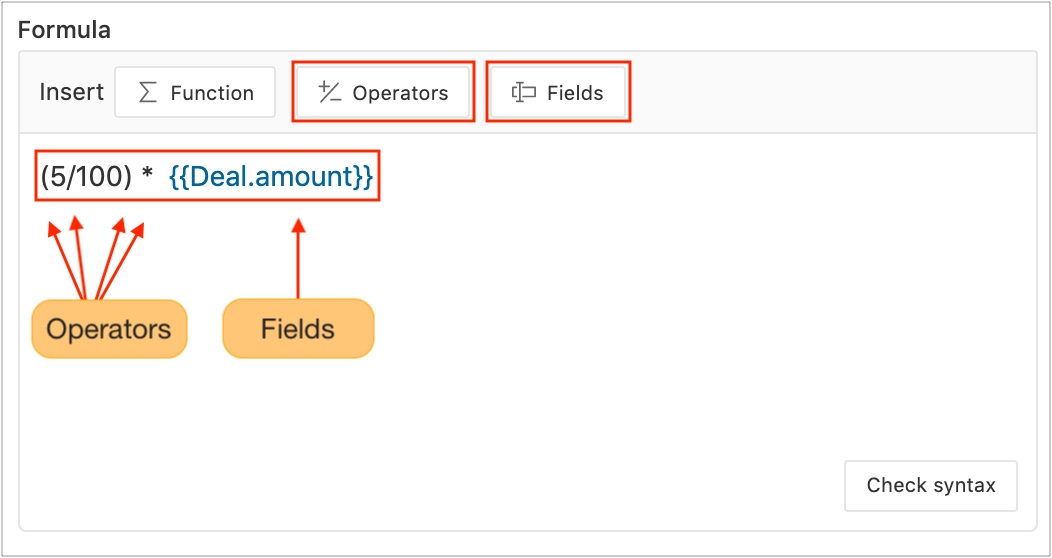Step 5: Check the formula syntax

Once you’ve entered the formula, you can check the syntactic validity of your formula. The system checks if the output matches the set return type. It also checks if the field values are of the same type. To see if your formula is valid, click the “Check Syntax” option. If an error is detected, it will display an error message explaining what went wrong.Here is an example of an error message. It will indicate the entity in which the error is, in this case, the operator/function section of the formula. A common error that occurs when using functions is forgetting to add the closing parenthesis. This is explained by the error message.Step 6: Click save, and you’re done!

# 3. What happens when the field value used in the formula is empty?

Say, the salesperson hasn’t filled in the dependent field yet. Here’s an example, we’ve set the commission based on “Deal Value” and have not entered the value. The formula field “Commission based on Deal Value” will then show the value as 0.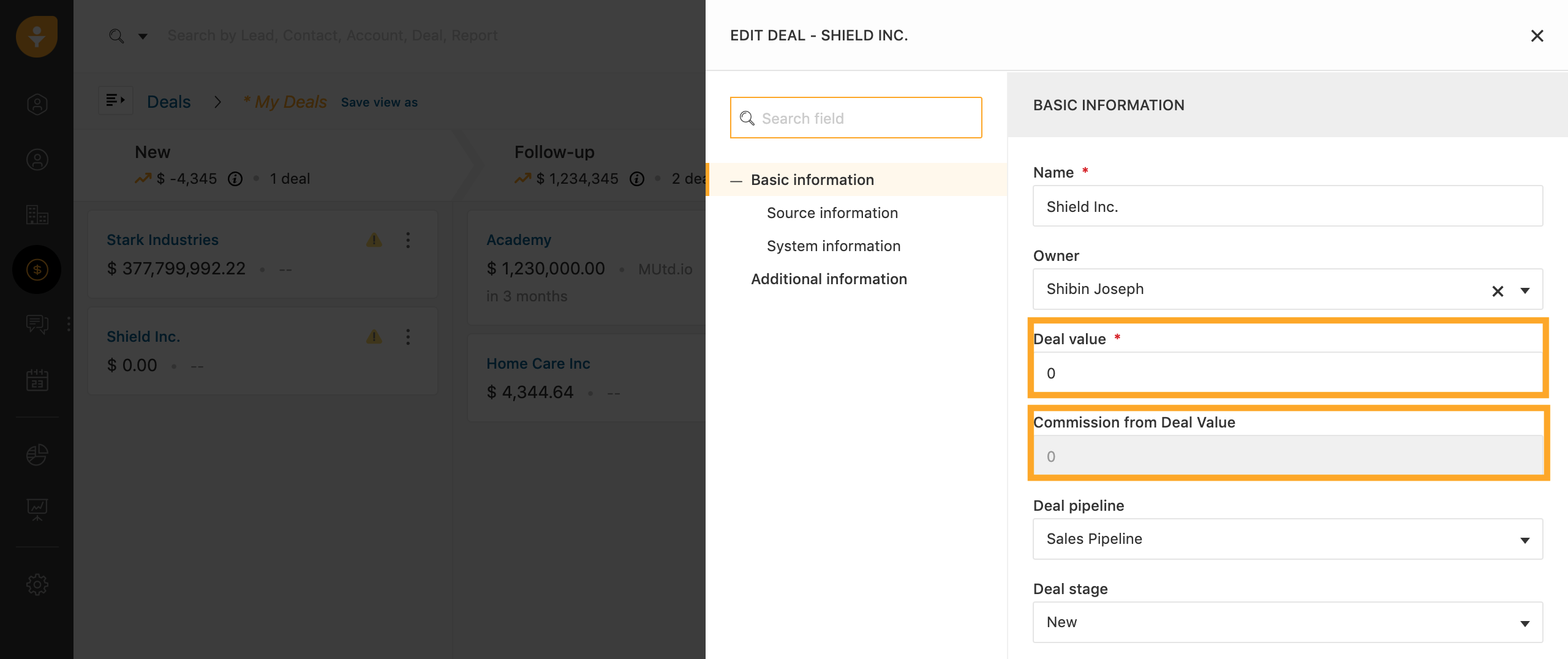In the case of text functions, the same message is displayed when the field is empty. In this example, we’ve set the formula for the “Trimmed Name” field to concatenate the first and last name. The first name has not been provided, so the trimmed field will display, “Enter value.”

# 4. What happens when I downgrade?

Formula fields are available to all users who are in plans Estate and Forest. In the case of a downgrade, your existing formula fields will not be deleted. The formula is removed, and the field becomes a manually calculated field. The user can enter the value based on their computation of the formula.

# 5. What are the supported formula field functions?

 MATHEMATICAL FUNCTIONS
 Function name Expression Description Example Max MAX(value1, value2) Returns the greater of two values. MAX( {{Deal.amount}} , {{Deal.base_currency_amount}} ) looks into the “Deal amount” and “Deal amount in base currency” field in your Freshsales and returns the highest value amongst the two. Min MIN(value1, value2) Returns the smaller of two values. MIN(Discount, 20) looks into the “Discount” field in your Freshsales and returns the lowest value (across all deals), while also considering the number 20. Floor FLOOR(value) Rounds down value to its nearest integer. FLOOR(23.8) is 23 Log LOG(value) Returns the logarithm of value, against a standard base viz. 2.718. LOG(5) is 1.43 Ceil CEIL(value) Rounds up a value to its nearest integer. CEIL(6.3) is 7 Square root SQRT(value) Returns the positive square root of a positive value. SQRT(225) is 15 Exponential function EXP(exponent) Raises Euler’s number 2.718 to a power i.e., 2.718^power. EXP(2) is 7.39 (calculated as 2.718^2). Power function POW(base, exponent) Raises a base value to a power. POW(3, 4) is 81, i.e. 3^4 = 81. Random random() Returns a random value between 0 and 1, where 0 is included and 1 is excluded. RAND() is 0.24. if if(logical_expression,value_if_true,value_if_false) Checks if the criteria set for a logical expression matches; executes conditions set for True if criteria matches, executes condition set for False if criteria fails. if( {{Deal.amount}} > 500, {{Deal.amount}} - ( {{Deal.amount}} * 20/100), {{Deal.amount}} - {{Deal.amount}} *5/100)Calculates discounted price based on Deal value. If Deal value is higher than 500, applies 20% discount.If Deal value is lower than 500, applies 5% discount.

 STRING FUNCTIONS
 FUNCTION NAME EXPRESSION DESCRIPTION EXAMPLE charAt charAt(‘text’, specified index) Pulls up a specified character from a piece of text. charAt(‘Deal size’, 2) is ‘a’. compareTo compareTo(‘text1’, ‘text2’) Compares two pieces of text based on their position in the dictionary. This function is case-sensitive. compareTo(‘deal’, ‘lead’) is -8, because “d” in “deal” comes 8 places before “l” in “lead” compareToIgnoreCase compareToIgnoreCase(‘text1’, ‘text2’) Compares two pieces of text based on their position in the dictionary. This function is not case-sensitive. compareToIgnoreCase(‘deal’, ‘DEAL’) is 0, because although there is a case difference, the characters are ultimately identical. CONCAT CONCAT(‘text1’, ‘text2’) Combines two pieces of text. CONCAT (‘First name’, ‘Last name’) combines values from both these fields to show the full name of a prospect. endsWith endsWith(‘text’, ‘specified characters’) Checks if a piece of text ends with specified characters; returns True if it ends and returns False if it doesn’t. This function is case-sensitive. endsWith(‘Lead stage’, ‘age’) is True. equals equals(‘text1’, ‘text2’) Checks if two pieces of text match; returns True if they match and returns False if they don’t. This function is case-sensitive. equals(‘leads’, ‘leads’) returns True. equalsIgnoreCase equalsIgnoreCase(‘text1’, ‘text2’) Checks if two pieces of text match; returns True if they match and returns False if they don’t. This function is not case-sensitive. equalsIgnoreCase(‘leads’, ‘LEADS’) returns True. indexOf indexOf(‘text’, ‘specified character’, fromIndex) Returns the index for a specified character in a piece of text, the first time it occurs. indexOf(‘pipeline’, ‘i’, 0) returns 1. This means in “pipeline”, you’re looking for the first occurrence of the character “i”, starting from index 0 i.e. starting from “p.” lastIndexOf lastIndexOf(‘text’, ‘specified character’, fromIndex) Returns the index for a specified character in a piece of text, the last time it occurs. Search runs backwards. lastIndexOf(‘pipeline’, ‘i’, 6) returns 5. This means in “pipeline”, you’re looking for the last occurrence of the character “i”, starting backwards from index 6 i.e. starting backwards from “n.” length length(‘text’) Returns the number of characters in a piece of text. len(‘John Wayne’) is 10. replace replace(‘text’, ‘oldCharacter’, ‘newCharacter’) Replaces a character every time it occurs in the text. replace('lead', 'l', 'L') replaces "lead" with "Lead."